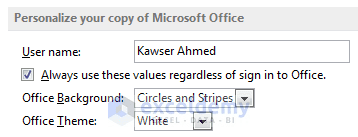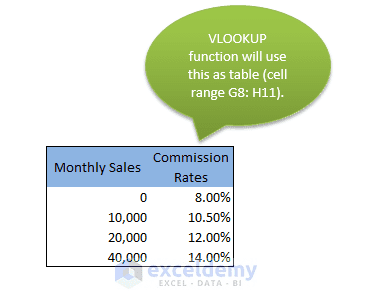Disclosure: This post may contain affiliate links, meaning when you click the links and make a purchase, we receive a commission.

# A detailed analysis of Excel VBA Function Procedure Arguments

We knew how create custom VBA functions and use them in the worksheet. This article focuses on various kinds of arguments you can use in VBA functions. Function arguments can be variables (including arrays), constants, literals, or expressions. Keep in mind the following about function procedure arguments:

• Some functions do not have any arguments.
• Some functions have a fixed number of required arguments (from 1 to 60).
• Some functions have a combination of required and optional arguments.

The following sections demonstrate how to use arguments effectively with functions. I didn’t cover optional arguments in this article.

## A VBA function with no argument

Though most VBA functions use arguments, that’s not a requirement. Excel has some predefined functions that don’t use any arguments, such as RAND(), TODAY(), and NOW().Choose File ➪ Options ➪ General to see this section.

The following is a simple example of a custom function that has no arguments. This function returns the UserName property of the Application object. This name appears in the Personalize your copy of Microsoft Office section of the Excel Options dialog box. This function is very simple, but it is the only way you can get the username to use in a worksheet cell or formula.

```Function OfficeUserName()
'Returns the name of the current user
End Function
```

When you enter the following formula into a worksheet cell, the cell displays the name of the current user:

When you use a function with no arguments, you must include a set of empty parentheses.

Read More: An Excel VBA function with two arguments

## A function with one argument

Before entering into details, at first, I introduce you to the method Speak(). This method has the following syntax:

expression.Speak (Text, [SpeakAsync], [SpeakXML], [Purge])

The expression represents a Speech object.

Though Speak() method can take three arguments, but Text argument is the required one, the rest are optional. I shall use the Speak() function by passing only Text argument. So, for this case, it is a function with one argument. This method uses Excel text-to-speech generator to “speak” the text passed to it through Text argument.

```Function SpeakTheText(text)
Application.Speech.Speak (text)
End Function
```

Note: To hear the voice, your system must be set up to play sound.

For example, if you want to use the SpeakTheText() function to hear the content of cell A1, you have to use the following formula:

`=SpeakTheText(A1)`

You can use this function in a slightly more complex formula, as shown below. In this example, the argument is a text string (“Good achievement”) rather than a cell reference.

`=IF(SUM(A:A)&gt;1000, SpeakTheText("Goal achievement"),)`

The above formula calculates the sum of the values in Column A. When that sum exceeds 1,000, you will hear “Good achievement”.

When you use the SpeakTheText function in a worksheet formula, the function always returns 0, because this function is returning nothing. In other words, I can say, there is no value assigned to the function’s name (SpeakTheText) variable.

Read More: An Excel VBA function with a range argument

### Another example of VBA function with one argument

Okay, now I’m going to deal with a more complex function. This function is designed for a sales manager who calculates monthly commission that he has to pay to his salespersons. To boost up the sales of the company, the commission rate structure is based on the amount sold— those who will sell more will earn a higher commission rate.

The function takes the sales amount through the only argument (Sales) and returns the commission earned by a salesperson. The function will calculate the commission according to the following table:

### Table: Monthly Sales Target & Commission Rates

Monthly SalesCommission Rate
0-\$9,9998.0%
\$10,000-\$19,99910.5%
\$20,000-\$39,99912.0%
\$40,000+14.0%

You can use more than one method to calculate commissions for various sales amounts.

### Using nested IF function

Assume that you have the sales amount for a particular salesperson in a worksheet cell A4. Now you can use the following formula:

```=IF (AND (A4>=0, A4<=9999.99), A4*0.08, IF (AND (A4>=10000, A4<=19999.99), A4*0.105, IF (AND (A4>=20000, A4<=39999.99), A4*0.12, IF (A4>=40000, A4*0.14, 0))))
```

This approach isn’t a good solution for the following reasons:

• Firstly, the formula is undoubtedly complex and not easy to understand.
• Secondly, the commission rates are hard-coded into the formula. If the commission structure changes in future, it would be tough and toilsome to modify the formula.

### Using VLOOKUP function

A simple and better solution is using a VLOOKUP table. I have entered the data of the above table (Monthly Sales Target & Commission Rates) in cell range G8: H11. Click this link to know about VLOOKUP function, if you don’t know.VLOOKUP table for the data that calculates the commission rates for sales target.

Note: It is important to note that I have used 0 for the data range 0-9999, 10,000 for 10,000-19,999, 20,0000 for 20,000-39,999 and 40,000 for 40,000+. When I do so, Excel searches data < 10,000 in 0 row, 10,000 ≤ data ≤ 19,999 in 10,000 row, 20,000 ≤ data ≤ 39,999 in row 20,000 and data ≥ 40,000 in row 40,000.

`=VLOOKUP(A4, \$G\$8: \$H\$11, 2)*A4`

Use this function in other cells to calculate the sales commission earned by the sales force.

### Using Custom function

Like the following one, you can create a custom function to calculate the commission earning by the sales force.

```Function CommissionEarning(Sales)
'Calculates sales commissions
Rate1 = 0.08
Rate2 = 0.105
Rate3 = 0.12
Rate4 = 0.14
Select Case Sales
Case 0 To 9999.99
CommissionEarning = Sales * Rate1
Case 10000 To 19999.99
CommissionEarning = Sales * Rate2
Case 20000 To 39999.99
CommissionEarning = Sales * Rate3
Case Is &gt;= 40000
CommissionEarning = Sales * Rate4
End Select
End Function
```

I have used the custom function in cell D4 using the argument A4, as A4 holds the sales. Then I have copied this function for other cells. The figure below shows this:

## A function with two arguments

In this example, I am going to write a VBA function that will calculate the area of a rectangle. We know the area of a rectangle= Length ☓ Width.

This is a very simple function. Below shows the code:

```Function Area(Length, Width)
'Finding out the Area of the Rectangle.
Area = Length * Width
MsgBox "The Area of your Rectangle is: " &amp; Area
End Function
```

This function takes two arguments(Length and Width) from the user and shows the area of the rectangle in a message box.

## A function with a range argument

Well, the last type of argument is the range. It is demonstrated here.

Let’s make some assumption. Say, you have some values. The number of values may be 100, 1000, or any. Your boss has told you to give him the average of largest 5 values of this data. What can you do? First, you’ll think that whether there is any predefined function in Excel to solve it efficiently. Yes, it has the LARGE function. The LARGE function does not give the result directly. You have to use it trickily. You can use the LARGE function in the following way (assuming that you have 20 values and the data range is named “DataAvg”):

```=(LARGE(DataAvg, 1)+LARGE(DataAvg, 2)+LARGE(DataAvg, 3)+
LARGE(DataAvg, 4)+LARGE(DataAvg, 5))/5```

The above formula will return the average of the largest 5 values of your data. The formula works fine, but you will face a problem when in next time your boss will ask you to average of 100 values from a data range of say 2000 values. This time you have to rewrite this formula in a new way and it is really toilsome. Here comes the custom VBA function, TopAverage (DatAvg, Num).

I have written the TopAverage function in the following way:

```Function TopAverage(DataAvg, Num)
'Returns the average of the highest Num values in Data
Sum = 0
For i = 1 To Num
Sum = Sum + WorksheetFunction.Large(DataAvg, i)
'Large(Data, i) returns the ith largest value from the Data.
Next i
TopAverage = Sum / Num
MsgBox "The average is: " &amp; TopAverage
End Function
```

This situation is an example of how a custom function can make things easier for you. This function takes two arguments: DataAvg and Num. DataAvg represents the range of data that you’re working with. Num is the number of largest values you want to average from the data range.

Read More: An Excel VBA function with one argument

The code initializes the Sum variable to 0. Then it uses For- Next loop to calculate the sum of the Num number largest values. Notice that I have used the built-in LARGE function of Excel in the code. You can use any worksheet function in the custom VBA function using WorksheetFunction and a period before the function name (WorksheetFunction.LARGE).

Finally, the average is found just dividing the Sum by the Num (Sum/Num). I showed the result in a message box with this text: “The average is: “.